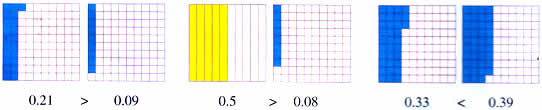Thursday , July 7 2022# NCERT 5th Class (CBSE) Mathematics: Decimals

### Comparing DecimalAdding and subtracting decimal numbers is just like adding and subtracting whole numbers. Remember to line up the decimal points one below the other. Change to like decimals if required.

(a) 5.32+3.8

5.32+3.8 = 9.12

(b) 0.96+1.2

0.96+1.2 = 2.16

(c) 2.1-0.87

2.1-0.87 = 1.23

(d) 1-0.4

1.00-0.4 = 0.58

## MULTIPLICATION OF DECIMALS

### A. Multiplying Decimals by a Whole Number

(a) A greeting card costs Rs 8.25. If Shabnam buys three cards, how much should she pay the shopkeeper?

8.25+8.25+8.25 = ?

or

3 × 8.25 = ?

Step 1. Multiply as with whole numbers ignoring the decimal.

8.25×3 = 2475

Step 2. Count the number of decimal places in the factors.

8.25 (2 decimal places) × 3 (0 decimal place) = 2475

Step 3. Show the number of decimal places in the product.

8.25×3 = 24.75 ( 2+0 = 2 decimal places inn all)

Answer: Shabnam has to pay Rs 24.75.

(b) 4.5×16 = 720 → 4.5×16 = 72.0

(c) 0.09×24 = 216 →  0.09×24 = 2.16

## चुनौती हिमालय की 5th NCERT CBSE Hindi Rimjhim Ch 18

चुनौती हिमालय की 5th Class NCERT CBSE Hindi Book Rimjhim Chapter 18 प्रश्न: लद्दाख जम्मू-कश्मीर राज्य में …